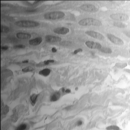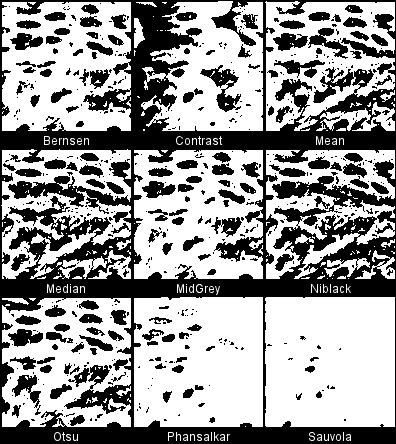# Auto Local ThresholdThe content of this page has not been vetted since shifting away from MediaWiki. If you’d like to help, check out the how to help guide!

This plugin binarises 8-bit images using various local thresholding methods. By ‘local’ here is meant that the threshold is computed for each pixel according to the image characteristings within a window of radius r (in pixel units) around it. The segmented phase is always shown as white (255).

For global thresholding rather than local, see the Auto Threshold plugin.

## Installation

ImageJ: requires v1.42m or newer. Download Auto_Threshold-X.Y.Z.jar and copy it into the ImageJ/plugins folder and either restart ImageJ or run the HelpUpdate Menus command. After this a new command should appear in ImageAdjustAuto Local Threshold.

Fiji: this plugin is part of the Fiji distribution, there is no need to download it.

## Use

Method selects the algorithm to be applied (detailed below).

The radius sets the radius of the local domain over which the threshold will be computed.

White object on black background sets to white the pixels with values above the threshold value (otherwise, it sets to white the values less or equal to the threshold).

Special parameters 1 and 2 sets specific values for each method. Those are detailed below for each method.

It you are processing a stack, one additional option is available: Stack can be used to process all the slices.

## Available methods

### Try all

Which method segments your data best? You can attempt to answer this question using the Try all option. This produces a montage with results from all the methods, so one can explore how the different algorithms perform on an image or stack.Original imageTry all methods.

When processing stacks with many slices, the montages can become very large (several times the original stack size) and one risks running out of ram. A popup window will appear (when stacks have more than 25 slices) to confirm whether the procedure should display the stack montages.

### Bernsen

Implements Bernsen’s thresholding method. Note that this implementation uses circular windows instead of rectangular in the original.

Parameter 1: is the contrast threshold. The default value is 15. Any number different than 0 will change the default value.

Parameter 2: not used, ignored.

The method uses a user-provided contrast threshold. If the local contrast (max-min) is above or equal to the contrast threshold, the threshold is set at the local midgrey value (the mean of the minimum and maximum grey values in the local window). If the local contrast is below the contrast threshold the neighbourhood is considered to consist only of one class and the pixel is set to object or background depending on the value of the midgrey.

``````if ( local_contrast < contrast_threshold )
pixel = ( mid_gray >= 128 ) ? object :  background
else
pixel = ( pixel >= mid_gray ) ? object : background
``````

doi:10.4236/oalib.1101203

doi:10.1117/1.1631315

Based on ME Celebi’s fourier_0.8 routines 1 and 2.

### Contrast

Based on a simple contrast toggle. Sets the pixel value to either white (255) or black (0) depending on whether its current value is closest to the local maximum or minimum respectively. The procedure is an extreme case of Toggle Contrast Enhancement, see for example:

doi:10.1007/978-3-662-05088-0

This procedure does not have user-provided parameters other than the kernel radius.

### Mean

This selects the threshold as the mean of the local greyscale distribution. A variation of this method uses the mean - C, where C is a constant.

``````pixel = ( pixel > mean - c ) ? object : background
``````

Parameter 1: is the C value. The default value is 0. Any other number will change the default value.

Parameter 2: not used, ignored.

### Median

This selects the threshold as the median of the local greyscale distribution. A variation of this method uses the median - C, where C is a constant.

``````pixel = ( pixel > median - c ) ? object : background
``````

Parameter 1: is the C value. The default value is 0. Any other number will change the default value.

Parameter 2: not used, ignored.

### MidGrey

This selects the threshold as the mid-grey of the local greyscale distribution (i.e. (max + min)/2. A variation of this method uses the mid-grey - C, where C is a constant.

``````pixel = ( pixel > ( ( max + min ) / 2 ) - c ) ? object : background
``````

Parameter 1: is the C value. The default value is 0. Any other number will change the default value.

Parameter 2: not used, ignored.

### Niblack

Implements Niblack’s thresholding method:

``````pixel = ( pixel >  mean + k * standard_deviation - c) ? object : background
``````

Parameter 1: is the k value. The default value is 0.2 for bright objects and -0.2 for dark objects. Any other number than 0 will change the default value.

Parameter 2: is the C value. This is an offset with a default value of 0. Any other number than 0 will change its value. This parameter was added in version 1.3 and is not part of the original implementation of the algorithm. The original algorithm is applied when C = 0.

Invalid citation arguments: `{"last"=>"Niblack", "first"=>"W", "year"=>"1986", "journal"=>"", "title"=>"An introduction to Digital Image Processing, Prentice-Hall"}`

Ported from ME Celebi’s fourier_0.8 routines 3 and 4.

### Otsu

Implements a local version of Otsu’s global threshold clustering. The algorithm searches for the threshold that minimizes the intra-class variance, defined as a weighted sum of variances of the two classes. The local set is a circular ROI and the central pixel is tested against the Otsu threshold found for that region.

doi:10.1109/TSMC.1979.4310076

Ported from C++ code by Jordan Bevik.

### Phansalkar

This is a modification of Sauvola’s thresholding method to deal with low contrast images.

doi:10.1109/ICCSP.2011.5739305

In this method, the threshold t is computed as:

``````t = mean * (1 + p * exp(-q * mean) + k * ((stdev / r) - 1))
``````

where mean and stdev are the local mean and standard deviation respectively. Phansalkar recommends k = 0.25, r = 0.5, p = 2 and q = 10. In this plugin, k and r are the parameters 1 and 2 respectively, but the values of p and q are fixed.

Parameter 1: is the k value. The default value is 0.25. Any other number than 0 will change its value.

Parameter 2: is the r value. The default value is 0.5. This value is different from Sauvola’s because it uses the normalised intensity of the image. Any other number than 0 will change its value.

Implemented from Phansalkar’s paper description, although this version uses a circular rather than rectangular local window.

### Sauvola

Implements Sauvola’s thresholding method, which is a variation of Niblack’s method

``````pixel = ( pixel > mean * ( 1 + k * ( standard_deviation / r - 1 ) ) ) ? object : background
``````

Parameter 1: is the k value. The default value is 0.5. Any other number than 0 will change the default value.

Parameter 2: is the r value. The default value is 128. Any other number than 0 will change the default value

doi:10.1016/S0031-3203(99)00055-2

Ported from ME Celebi’s fourier_0.8 routines 5 and 6.